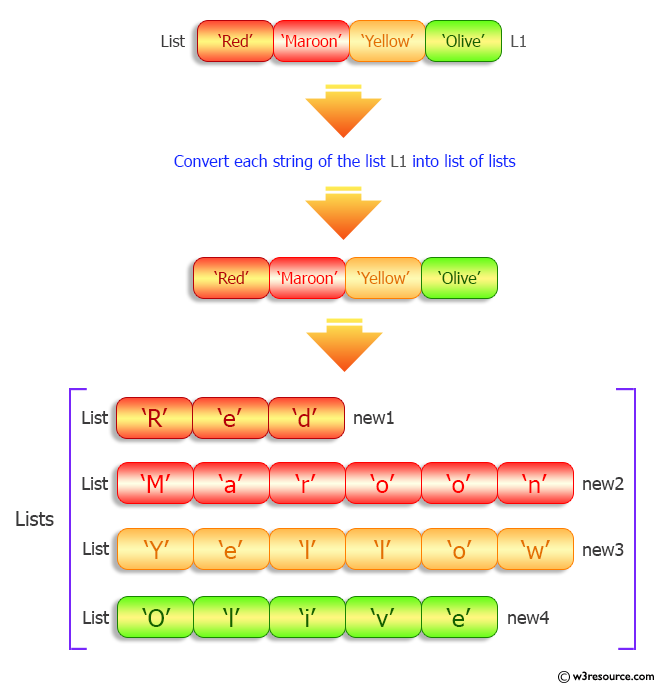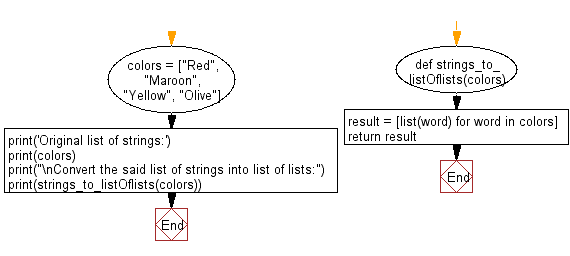﻿ Python: Convert a given list of strings into list of lists - w3resource# Python: Convert a given list of strings into list of lists

## Python List: Exercise - 167 with Solution

Write a Python program to convert a given list of strings into list of lists.

Sample Solution:

Python Code:

``````def strings_to_listOflists(colors):
result = [list(word) for word in colors]
return result

colors = ["Red", "Maroon", "Yellow", "Olive"]
print('Original list of strings:')
print(colors)
print("\nConvert the said list of strings into list of lists:")
print(strings_to_listOflists(colors))
```
```

Sample Output:

```Original list of strings:
['Red', 'Maroon', 'Yellow', 'Olive']

Convert the said list of strings into list of lists:
[['R', 'e', 'd'], ['M', 'a', 'r', 'o', 'o', 'n'], ['Y', 'e', 'l', 'l', 'o', 'w'], ['O', 'l', 'i', 'v', 'e']]
```

Pictorial Presentation:Flowchart:## Visualize Python code execution:

The following tool visualize what the computer is doing step-by-step as it executes the said program:

Python Code Editor:

Have another way to solve this solution? Contribute your code (and comments) through Disqus.

What is the difficulty level of this exercise?

Test your Python skills with w3resource's quiz

﻿

## Python: Tips of the Day

Floor Division:

When we speak of division we normally mean (/) float division operator, this will give a precise result in float format with decimals.

For a rounded integer result there is (//) floor division operator in Python. Floor division will only give integer results that are round numbers.

```print(1000 // 300)
print(1000 / 300)```

Output:

```3
3.3333333333333335```